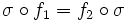# Function property

(diff) ← Older revision | Latest revision (diff) | Newer revision → (diff)
The function property must satisfy isomorphism-invariance: if$f_1:G \to G$ and$f_2:H \to H$ are functions, and there is an isomorphism$\sigma:G \to H$ such that$\sigma \circ f_1 = f_2 \circ \sigma$, then$f_1$ satisfies the function property iff$f_2$ satisfies the function property.